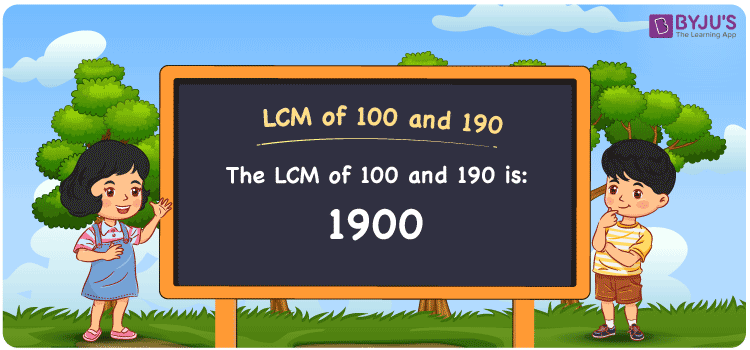# LCM of 100 and 190

LCM of 100 and 190 is 1900. The LCM is the method to find the least common multiple between any two or more numbers. Prime Factorisation and Division Method for LCM and HCF is a vital study material for the students which helps them to grasp the concept thoroughly. Time management skills can be improved among the students by practising the problems using this article prepared by experts. Let us learn the method of finding the least common multiple of 100 and 190 using suitable examples and FAQs here.

## What is LCM of 100 and 190?

The Least Common Multiple of 100 and 190 is 1900.## How to Find LCM of 100 and 190?

LCM of 100 and 190 can be obtained by using three methods:

• Prime Factorisation
• Division method
• Listing the Multiples

### LCM of 100 and 190 Using Prime Factorisation Method

In the prime factorisation method, LCM of 100 and 190 can be obtained by multiplying prime factors raised to their respective highest power.

100 = 2 × 2 × 5 × 5

190 = 2 × 5 × 19

LCM (100, 190) = 2 × 2 × 5 × 5 × 19 = 1900

### LCM of 100 and 190 Using Division Method

In the division method, to calculate the least common multiple of 100 and 190, we divide the numbers 100 and 190 by their prime factors until we get the result as one in the complete row. The product of these divisors represents the least common multiple of 100 and 190.

 2 100 190 2 50 95 5 25 95 5 5 19 19 1 19 x 1 1

No further division can be done.

Hence, LCM (100, 190) = 2 × 2 × 5 × 5 × 19 = 1900

### LCM of 100 and 190 Using Listing the Multiples

In this method, we list down the multiples of 100 and 190 to find the least common multiple among them. The following list shows the multiples of 100 and 190.

Multiples of 100: 100, 200, 300, 400, 500, 600, 700, 800, 900, 1000, ……, 1700, 1800, 1900,….

Multiples of 190: 190, 380, 570, 760, 950, 1140, 1330, 1520, 1710, 1900, ………

LCM (100, 190) = 1900

## Related Articles

Least Common Multiple (LCM)

LCM of Two Numbers

HCF and LCM

LCM calculator

LCM with Examples

LCM Formula

## Video Lesson on Applications of LCM## Solved Examples

1. What is the smallest number that is divisible by both 100 and 190?

Solution: 1900 is the smallest number that is divisible by both 100 and 190.

2. The GCD and LCM of the two numbers are 10 and 1900. If one number is 100, what is the other number?

Solution: Let the other number be k

We know that,

GCD × LCM = 100 × k

k = (GCD × LCM) / 100

k = (10 × 1900) / 100

k = 190

Hence the other number is 190.

## Frequently Asked Questions on LCM of 100 and 190

### What is the LCM of 100 and 190?

The LCM of 100 and 190 is 1900.

### Is the LCM of 100 and 190 the same as the HCF of 100 and 190?

No. The LCM of 100 and 190 is 1900 and the HCF of 100 and 190 is 10.

### Name the methods used to find the LCM of 100 and 190.

The methods used to find the LCM of 100 and 190 are as follows:

Prime Factorisation

Division method

Listing the Multiples

### Mention the relation between the GCF and LCM of 100 and 190.

The relation between the GCF and the LCM of 100 and 190 is

GCF × LCM = 100 × 190

GCF × LCM = 19000

### Determine the GCF if the LCM of 100 and 190 is 1900.

GCF × LCM = 100 × 190

Given

LCM = 1900

GCF × 1900 = 100 x 190

GCF = 10